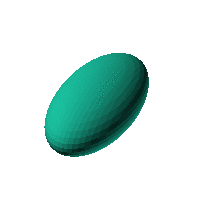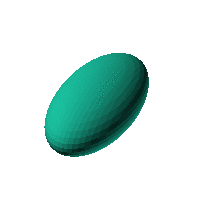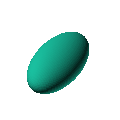Blob Farm# blob68The blob in all its glory:A nice simple ellipsoid.

 Click on the snapshot to download the blob's stl file.Octave Code:
```  # name of the blob
project = "blob68";

# function at origin must be <0, and >0 far enough away.  w=0 defines the surface
function w = f(x2,y2,z2,c,r)
x = (x2-c(1))/r(1);
y = (y2-c(2))/r(2);
z = (z2-c(3))/r(3);

w = x.^2+y.^2+z.^2-1.0;  # <<<<<<<<<<<  THE BLOB FUNCTION

endfunction;

step = 2;  # grid pitch in mm  start with 4mm to see the shape quickly.  Once you have it just right, change to 2mm for printing

# use the default prewarp function
# use default grid extents (xmin, xmax, etc)

c_outer = [0,0,0];
r_outer = [20,40,15]; # this is why it will not just be a sphere

source("../octave/func2stl_v01.m");  # do all the calculations
```
GNU Octave